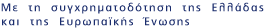Now showing items 1-20 of 34

• #### Analytical lattice Boltzmann solutions for thermal flow problems ﻿

(2003)
Analytical solutions based on two 13-bit (hexagonal and square) and one 17-bit square lattice Boltzmann BGK models have been obtained for the Couette flow, with a temperature gradient at the boundaries. The analytical ...
• #### The application of complex network time series analysis in turbulent heated jets ﻿

(2014)
In the present study, we applied the methodology of the complex network-based time series analysis to experimental temperature time series from a vertical turbulent heated jet. More specifically, we approach the hydrodynamic ...
• #### Application of the integro-moment method to steady-state two-dimensional rarefied gas flows subject to boundary induced discontinuities ﻿

(2008)
The computational efficiency of the integro-moment method for solving steady-state two-dimensional rarefied gas flows is investigated. The two-dimensional boundary driven flow of a single gas in a cavity is used as a model ...
• #### Complex Dynamics in Non-linear Real Estate Models ﻿

(2013)
In the present paper we study the complex dynamics of non-linear cobweb models of real estate markets in discrete time. Our model incorporates two sub-markets, land and housing, interacting with each other. We start by ...
• #### Computer aided analysis of viscous film flow along an inclined wavy wall ﻿

(1999)
The steady, laminar flow of a Newtonian liquid along an inclined wavy wall is studied in a two-dimensional numerical experiment using the Galerkin finite element method. The dimensionless Navier-Stokes equations are solved ...
• #### Conditional symmetries and the canonical quantization of constrained minisuperspace actions: The Schwarzschild case ﻿

(2013)
A conditional symmetry is defined, in the phase space of a quadratic in velocities constrained action, as a simultaneous. conformal symmetry of the supermetric and the superpotential. Iris proven that such a symmetry ...
• #### Decoupling of the general scalar field mode and the solution space for Bianchi type I and V cosmologies coupled to perfect fluid sources ﻿

(2006)
The scalar field degree of freedom in Einstein's plus matter field equations is decoupled for Bianchi type I and V general cosmological models. The source, apart from the minimally coupled scalar field with arbitrary ...
• #### Detection of low-dimensional chaos in wind time series ﻿

(2009)
In the present work we investigated the existence of low-dimensional deterministic chaos in wind time series. The time series were obtained from the New Anchialos (Greece) Air Base Measurement station. In a first place we ...
• #### Dynamo transition in low-dimensional models ﻿

(2008)
Two low-dimensional magnetohydrodynamic models containing three velocity and three magnetic modes are described. One of them (nonhelical model) has zero kinetic and current helicity, while the other model (helical) has ...
• #### Effects of wall roughness on flow in nanochannels ﻿

(2009)
Nonequilibrium molecular dynamics simulation is applied to investigate the effect of periodic wall roughness on the flow of liquid argon through krypton nanochannels. The effect of the length of a rectangular protrusion ...
• #### Energy and momentum distributions of the magnetic solution to (2+1) Einstein-Maxwell gravity ﻿

(2005)
We use Moller's energy-momentum complex in order to explicitly evaluate the energy and momentum density distributions associated with the three-dimensional magnetic solution to the Einstein-Maxwell equations. The magnetic ...
• #### Energy Distribution of a Regular Black Hole Solution in Einstein-Nonlinear Electrodynamics ﻿

(2015)
A study about the energy momentum of a new four-dimensional spherically symmetric, static and charged, regular black hole solution developed in the context of general relativity coupled to nonlinear electrodynamics is ...
• #### A fast iterative model for discrete velocity calculations on triangular grids ﻿

(2010)
A fast synthetic type iterative model is proposed to speed up the slow convergence of discrete velocity algorithms for solving linear kinetic equations on triangular lattices. The efficiency of the scheme is verified both ...
• #### Feedback controlling against chaos ﻿

(2000)
A P-D (Proportional plus Derivative) feedback controller is proposed for the control of chaotic dynamic systems. Using this feedback law the design requirement of command matching is satisfied independently of the perturbations ...
• #### Formulation and stability analysis of rapidly convergent iteration schemes for the 2-D linearized BGK equation ﻿

(2007)
Various H-N rapidly convergent schemes are proposed to speed up the slow convergence of the iterative solution of 2-D linearized Bhatnagar-Gross-Krook (BGK) kinetic equation containing up to the first moments of the ...
• #### A homogenous random fractal model for time series produced by constant energy molecular dynamics simulations ﻿

(2003)
In the present work we present an analysis of time series of instantaneous temperature and pressure produced during microcanonical (constant energy) molecular dynamics (MD). Simulations were applied to a nickel oxide grain ...
• #### Interpolation based numerical procedure for solving two-point nonlinear boundary value problems ﻿

(2004)
The present paper offers a simple, efficient and accurate straightforward numerical method for solving two-point nonlinear boundary value problems (NBVPs), which are frequently encountered in a broad discipline of sciences. ...
• #### Lattice kinetic simulations in three-dimensional magnetohydrodynamics ﻿

(2004)
A lattice kinetic algorithm to simulate three-dimensional (3D) incompressible magnetohydrodynamics is presented. The fluid is monitored by a distribution function, which obeys a scalar kinetic equation, subject to an ...
• #### Locally homogeneous spaces, induced Killing vector fields and applications to Bianchi prototypes ﻿

(2012)
An answer to the question: Can, in general, the adoption of a given symmetry induce a further symmetry, which might be hidden at a first level? has been attempted in the context of differential geometry of locally homogeneous ...
• #### Measurements of liquid film flow as a function of fluid properties and channel width: Evidence for surface-tension-induced long-range transverse coherence ﻿

(2011)
We study experimentally the influence of the transverse dimension on film flow in relatively wide channels with sidewalls. Large deviations from two-dimensional predictions are observed in the primary instability and in ...